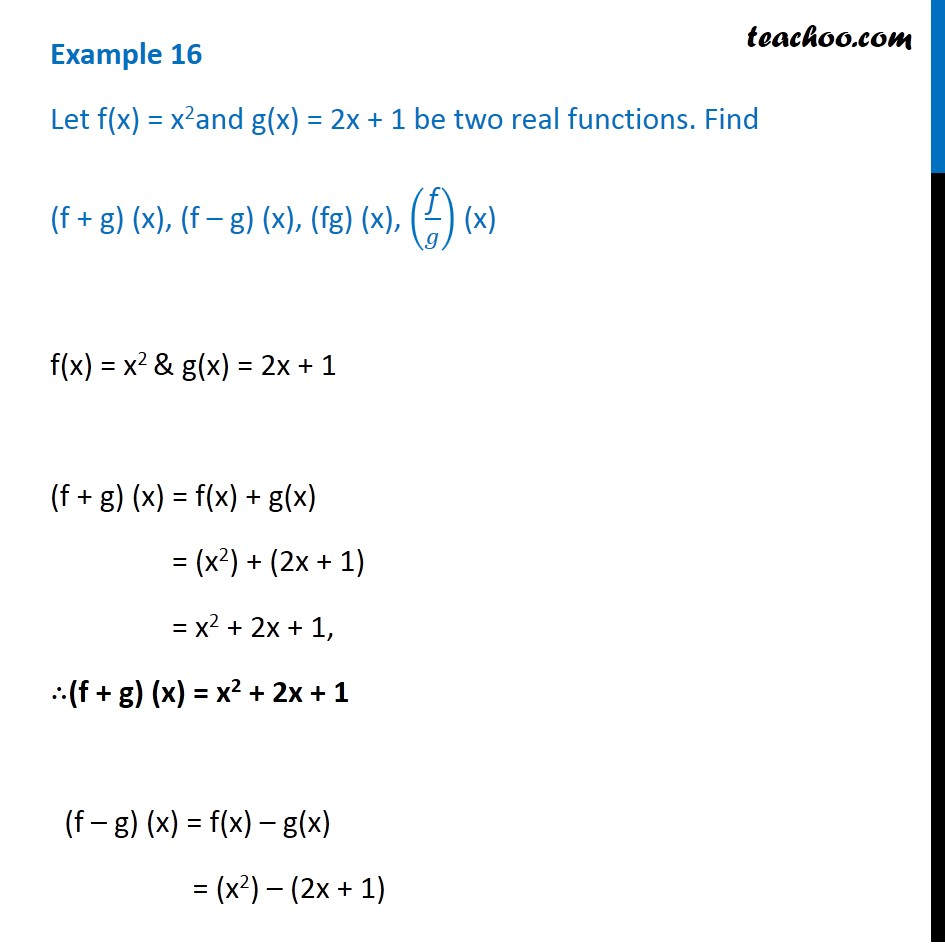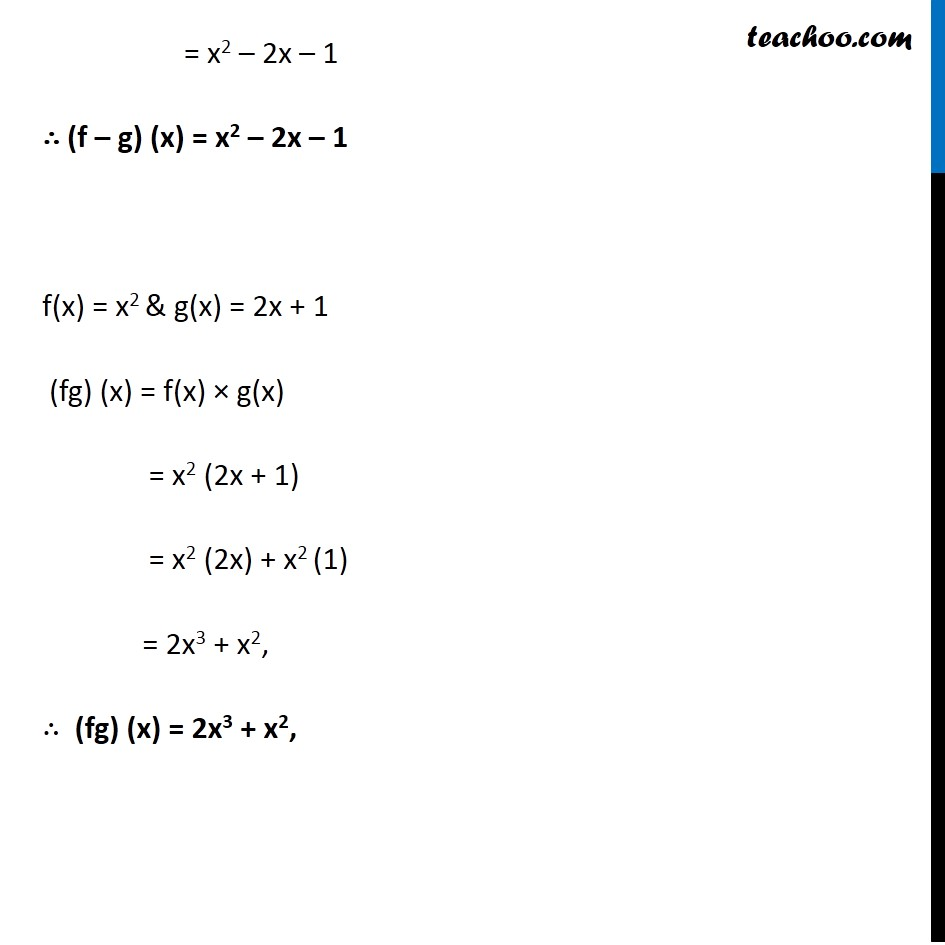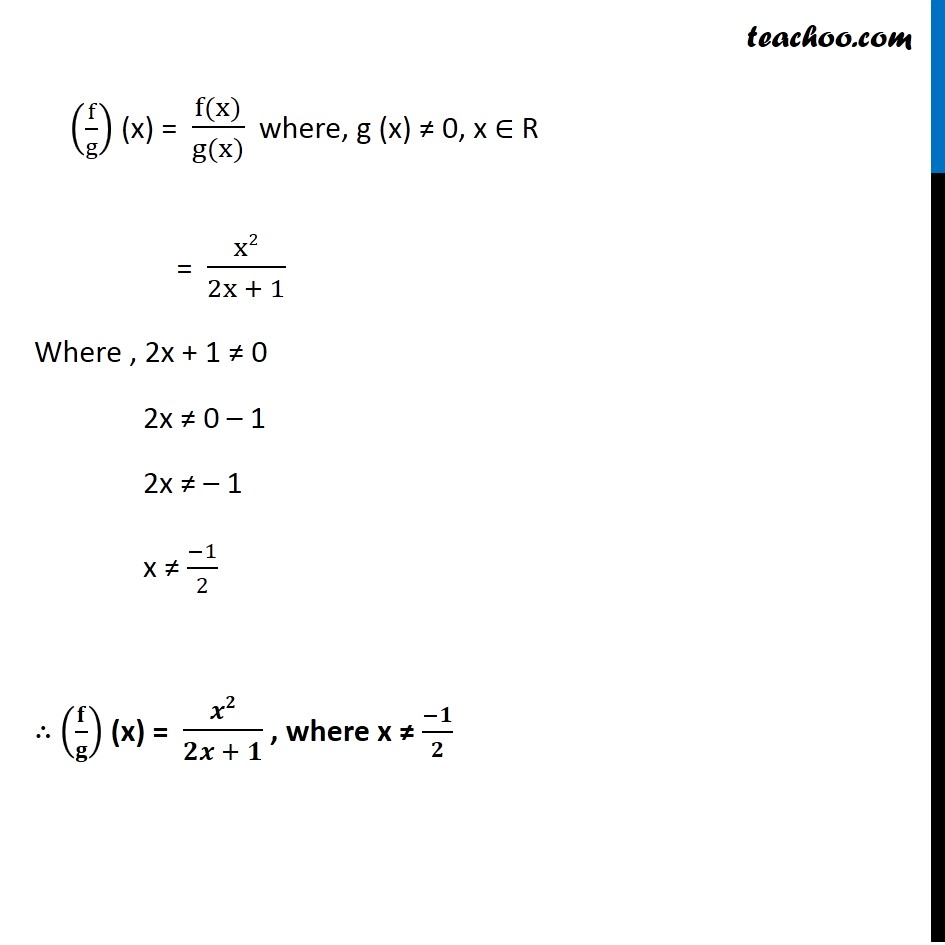1. Chapter 2 Class 11 Relations and Functions (Term 1)
2. Serial order wise
3. Examples

Transcript

Example 16 Let f(x) = x2and g(x) = 2x + 1 be two real functions. Find (f + g) (x), (f – g) (x), (fg) (x), ("f" /𝑔) (x) f(x) = x2 & g(x) = 2x + 1 (f + g) (x) = f(x) + g(x) = (x2) + (2x + 1) = x2 + 2x + 1, ∴(f + g) (x) = x2 + 2x + 1 (f – g) (x) = f(x) – g(x) = (x2) – (2x + 1) = x2 – 2x – 1 ∴ (f – g) (x) = x2 – 2x – 1 f(x) = x2 & g(x) = 2x + 1 (fg) (x) = f(x) × g(x) = x2 (2x + 1) = x2 (2x) + x2 (1) = 2x3 + x2, ∴ (fg) (x) = 2x3 + x2, (f/g) (x) = (f(x))/(g(x)) where, g (x) ≠ 0, x ∈ R = x2/(2x + 1) Example 16 Let f(x) = x2and g(x) = 2x + 1 be two real functions. Find (f + g) (x), (f – g) (x), (fg) (x), ("f" /𝑔) (x) f(x) = x2 & g(x) = 2x + 1 (f + g) (x) = f(x) + g(x) = (x2) + (2x + 1) = x2 + 2x + 1, ∴(f + g) (x) = x2 + 2x + 1 (f – g) (x) = f(x) – g(x) = (x2) – (2x + 1) = x2 – 2x – 1 ∴ (f – g) (x) = x2 – 2x – 1 f(x) = x2 & g(x) = 2x + 1 (fg) (x) = f(x) × g(x) = x2 (2x + 1) = x2 (2x) + x2 (1) = 2x3 + x2, ∴ (fg) (x) = 2x3 + x2, (f/g) (x) = (f(x))/(g(x)) where, g (x) ≠ 0, x ∈ R = x2/(2x + 1) Where , 2x + 1 ≠ 0 2x ≠ 0 – 1 2x ≠ – 1 x ≠ (−1)/2 ∴ (𝐟/𝐠) (x) = 𝒙𝟐/(𝟐𝒙 + 𝟏) , where x ≠ (−𝟏)/𝟐

Examples